## Social Question# Did you ever realize that long division is the reverse operation of multiplication?

Asked by LostInParadise (29005) February 1st, 2018

I have been tutoring an adult in arithmetic. In the process I noticed something that I never noticed before. It now seems so obvious.

Let me explain. Imagine multiplying two numbers. Let’s say that the bottom number has 3 digits. Going from right to left, you multiply each of the three digits with the top number. Then you add the three lines.

Now imagine dividing top number into the answer you got from the multiplication. Going from left to right (reverse of right to left), you produce each of the three digits of the bottom number, multiply and subtract (reverse of adding). Each line that you subtract is exactly the same as the corresponding line from the multiplication. When adding the lines in the multiplication, you go from zero (in a sense) to the product of the two numbers. When dividing, you subtract from the product to eventually get zero.

I have not gotten around yet to teaching division. I wonder if pointing out the above observation would be useful for teaching it.

Observing members: 0Composing members: 0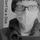Kinda always known this. However, by all means teach this. I took a grotesque amount of math in High School and College and I have tutored all three of my sisters kids in math for the last decade.

In high school I was mostly taught how.. In college I learned the “why”. The latter is a hell of a lot more valuable.

johnpowell (17878)“Great Answer” (2) Flag as…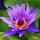Taught myself long division because I was out sick from school.

Tropical_Willie (28340)“Great Answer” (0) Flag as…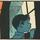And multiplication is repeated addition, and division is repeated subtraction (and subtraction is addition with negative numbers!).

@LostInParadise You have actually gained insight to the interconnectedness of mathematics. Mathematical operations are not separate and distinct, but rather intertwined and variations of each other.

It may help you express an overarching explanation of division when you get around to teaching it.

zenvelo (36782)“Great Answer” (4) Flag as…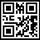Yes, we learned that when I was in fifth grade.

elbanditoroso (30572)“Great Answer” (3) Flag as…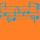It isn’t my go-to method for multiplication so I haven’t dwelled on it.. I don’t think it’s the best method from a pedagogical standpoint since it’s very procedural hardly conceptual. I prefer something like distribution. So 16×23 = (10+6)(20+3) = 200 + 30 + 120 + 18 = 368. Or if you can handle it, 16(20+3) = 320 + 48. I’m not generally good at doing arithmetic in my head in any way, and it’s nothing to sweat about.

But that is really cool. I never noticed that. I have been dividing polynomials lately, so I’m curious to see what this looks like when dealing with indeterminates.

dxs (15160)“Great Answer” (3) Flag as…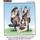No. Its still above my head. I use a calculator and my fingers to count.

RedDeerGuy1 (19445)“Great Answer” (1) Flag as…@dxs Dividing polynomials is an exercise in deconstructing products into factors. Once you get that down it will become a lot easier.

zenvelo (36782)“Great Answer” (2) Flag as…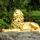I knew division was the opposite of multiplication conceptually, especially by some point when math classes started saying so, which was years after the math classes that had us learn long division techniques. But by that time, I almost never was using long division for much of anything.

Much later, tutoring children aggravated by public school math classes, I was quite horrified to see middle schoolers who were learning mainly to survive one type of exercise at a time, and to do it exactly as taught, with near-zero conceptual understanding of practically anything, including that division was the opposite or multiplication.

I think that what division and multiplication are in terms of tangible numbers or amounts of things ought to be taught solidly conceptually before working on arithmetic exercises. Otherwise it’s largely abstract torment for many students.

Zaku (27052)“Great Answer” (1) Flag as…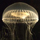Even I knew this. And I am a mathtard. That is, I suffer from mathtardation….

MrGrimm888 (16914)“Great Answer” (1) Flag as…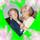Yes, I knew this as it was taught to us in elementary school. When we were learning our multiplication tables, we also learned that division was the “opposite” of multiplication. I have a vague memory of worksheets that had multiplication and division facts on them.

3×9 = 27
9×3 = ___
27/3 = 9
27/9 = ___

Or something like that.

tedibear (19070)“Great Answer” (1) Flag as…@zenvelo I don’t really have any difficulty dividing polynomials, I’m just looking for the analogy. In this case I’m thinking it’d be distribution being the opposite of factoring. That makes total sense.

On a side note, but actually not unrelated, a few months ago my friend and I were looking at simplifying an expression looking something like this: 4xy+7y. We immediately said what to do next at the same time—I said “factor” and my friend said “combine like terms.” At that moment I realized factoring and combining like terms must be the same process. Blew my mind, and I felt stupid trying to make sense out of it. Maybe it isn’t obvious, though.

dxs (15160)“Great Answer” (3) Flag as…